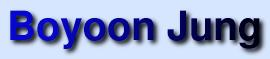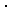Boyoon Jung's Library Manual

# bj::MultivariateGaussian Class Reference [Machine Learning Techniques]

A multivariate gaussian distribution. More...

`#include <gaussian.h>`

List of all members.

## Public Member Functions

MultivariateGaussian (int dimension, gsl_vector *mean=0, gsl_matrix *cov=0)
A constructor.
~MultivariateGaussian (void)
A destructor.
double p (gsl_vector *x, gsl_vector *mean, gsl_matrix *cov)
Compute the probability of a data point x with given mean and covariance.
double p (gsl_vector *x)
Compute the probability of a data point x.
gsl_vector * mean (void)
Get the current mean vector.
void setMean (gsl_vector *m)
Set a mean vector.
gsl_matrix * covariance (void)
Get the current covariance matrix.
void setCovariance (gsl_matrix *cov)
Set a covariance matrix.

## Detailed Description

A multivariate gaussian distribution.

## Constructor & Destructor Documentation

 bj::MultivariateGaussian::MultivariateGaussian ( int dimension, gsl_vector * mean = `0`, gsl_matrix * cov = `0` )

A constructor.

Parameters:
 dimension The dimension of data points. mean A mean vector. cov A covariance matrix.

 bj::MultivariateGaussian::~MultivariateGaussian ( void )
 A destructor.

## Member Function Documentation

 gsl_matrix* bj::MultivariateGaussian::covariance ( void ) ` [inline]`
 Get the current covariance matrix.

 gsl_vector* bj::MultivariateGaussian::mean ( void ) ` [inline]`
 Get the current mean vector.

 double bj::MultivariateGaussian::p ( gsl_vector * x )
 Compute the probability of a data point x.

 double bj::MultivariateGaussian::p ( gsl_vector * x, gsl_vector * mean, gsl_matrix * cov )
 Compute the probability of a data point x with given mean and covariance.

 void bj::MultivariateGaussian::setCovariance ( gsl_matrix * cov )
 Set a covariance matrix.

 void bj::MultivariateGaussian::setMean ( gsl_vector * m ) ` [inline]`
 Set a mean vector.

The documentation for this class was generated from the following file:
ResearchProjectsPublications

ToolsBjLib ManualPlayer/StagePyPlayerMicroStrain IMUGPS/DGPSParticle Filter

PersonalWelcomeCurriculum VitaeResumeContact

Outside LinksUSCUSC CS DeptCRESRobotics LabRESL